Home

# Wave dispersion in pulsar plasma. Part 2. Pulsar frame

## Abstract

Wave dispersion in a pulsar plasma is discussed emphasizing the relevance of different inertial frames, notably the plasma rest frame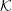${\mathcal{K}}$ and the pulsar frame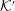${\mathcal{K}}^{\prime }$ in which the plasma is streaming with speed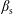$\unicode[STIX]{x1D6FD}_{\text{s}}$ . The effect of a Lorentz transformation on both subluminal,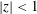$|z|<1$ , and superluminal,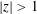$|z|>1$ , waves is discussed. It is argued that the preferred choice for a relativistically streaming distribution should be a Lorentz-transformed Jüttner distribution; such a distribution is compared with other choices including a relativistically streaming Gaussian distribution. A Lorentz transformation of the dielectric tensor is written down, and used to derive an explicit relation between the relativistic plasma dispersion functions in${\mathcal{K}}$ and${\mathcal{K}}^{\prime }$ . It is shown that the dispersion equation can be written in an invariant form, implying a one-to-one correspondence between wave modes in any two inertial frames. Although there are only three modes in the plasma rest frame, it is possible for backward-propagating or negative-frequency solutions in${\mathcal{K}}$ to transform into additional forward-propagating, positive-frequency solutions in${\mathcal{K}}^{\prime }$ that may be regarded as additional modes.

## References

Hide All
MathJax
MathJax is a JavaScript display engine for mathematics. For more information see http://www.mathjax.org.

# Wave dispersion in pulsar plasma. Part 2. Pulsar frame

## Metrics

### Full text viewsFull text views reflects the number of PDF downloads, PDFs sent to Google Drive, Dropbox and Kindle and HTML full text views.

Total number of HTML views: 0
Total number of PDF views: 0 *Loading metrics...

### Abstract viewsAbstract views reflect the number of visits to the article landing page.

Total abstract views: 0 *Loading metrics...

* Views captured on Cambridge Core between <date>. This data will be updated every 24 hours.

Usage data cannot currently be displayed.